# Plus Two Chemistry Chapter Wise Questions and Answers Chapter 9 Coordination Compounds

Students can Download Chapter 9 Coordination Compounds Questions and Answers, Plus Two Chemistry Chapter Wise Questions and Answers helps you to revise the complete Kerala State Syllabus and score more marks in your examinations.

## Kerala Plus Two Chemistry Chapter Wise Questions and Answers Chapter 9 Coordination Compounds

### Plus Two Chemistry Coordination Compounds One Mark Questions and Answers

Question 1.
The ions or molecules bound to the central atom/ion in the coordination entity are called ___________.
Ligands

Question 2.
In [Co(C2O4)3]3-, the coordination number of cobalt is _________.
six

Question 3.
Which complex has a square planar structure?
(a) [Ni(CO)4]
(b) [NiCI4]2-
(c) [Ni(H2O)6]2+
(d) [CU(NH3)4]2+
(d) [CU(NH3)4]2+

Question 4.
Say TRUE or FALSE.
[Co(NO3)(NH3)5]SO4 and [Co(NO3)(NH3)4(SO4)](NH3) are ionisation isomers.
FALSEQuestion 5.
The formula of Wilkinson’s catalyst is _________.
[RhCl(PPh3)3]

Question 6.
The charge of Ni in [Ni(CO)4] is
(a) +1
(b) +2
(c) 0
(d) +4
(c) 0

Question 7.
The central metal ion present in chlorophyll?
(a) Fe2+
(b) Cu2+
(c) Mg2+
(d) CO2+
(c) Mg2+

Question 8.
EDTA is a dentate ligand
(a) uni dentate
(b) bidentate
(c) TridentateQuestion 9.
Which is an example for homoleptic complexes
(a) [Co(NH3Cl2]+
(b) [CoNH3)6]3+
(c) [Cr(NH3)(H2O)3]Cl3
(d) [CoCl2(en)2]
(b) [CoNH3)6]3+

Question 10.
Ammonia will not form complex with
(a) Ag2+
(b) Pb2+
(c) Cu2+
(d) Cd2+
(e) Fe2+
(b) Pb2+

### Plus Two Chemistry Coordination Compounds Two Mark Questions and Answers

Question 1.
In a seminar, Jishnu argued that the “hexaflourocobaltate(III) ion is highly paramagnetic than hexacyanoferrate(III) ion.

1. Do you agree with this words?
2. Explain it.
3. Write the formulae of the given coordination compounds.

1. Yes
2. CN is a strong field ligand so paring occurs. Number of unpaired electron decreases, paramagnetism decreases. F is a weak field ligand so no paring occurs, paramagnetism increases.
3. [CoF6]3-and [Co(CN)6]3-Question 2.
Raju: Coordination compounds are coloured.
Ramu: No, co-ordination compounds are colourless.

1. Whose statement is correct?

1. Raju’s statement is correct. Coordination compounds are usually coloured.
2. The colour of coordination compounds is due to d-d transition.

Question 3.
Why is geometrical isomerism not possible in tetrahedral complexes having two different types of unidentate ligands coordinated with the central metal ion?
Tetrahedral complexes do not show geometrical isomerism because the relative positions of the unidentate ligands attached to the central metal atom are the same with respect to each other.

Question 4.
FeSO4 solution mixed with (NH4)2SO4 solution in 1:1 molar ratio gives the test of Fe2+ ion but CuSO4 solution mixed with aqueous ammonia in 1:4 molar ratio does not give the test of Cu2+ ion. Explain why?
FeSO4 does not form any complex with (NH4)2SO4. Instead, it forms a double salt FeSO4. (NH4)2SO4.6H2O which dissociates completely into ions. CuSO4 when mixed with NH3 forms a complex [CU(NH3)4]SO4 in which the complex ion [CU(NH3)4]2+ does not dissociate to give Cu2+ ion.

Question 5.
Write the geometrical isomers of [Pt(NH3)(Br)(Cl) (py)] and how many of these will exhibit optical isomerism?
The complex [Pt(NH3)(Br)(Cl)(py)] will form three geometrical isomers: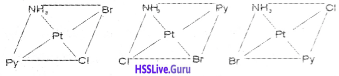Square planar complexes of this type will not show geometrical isomerism.Question 6.
Aqueous copper sulphate solution (blue in colour) gives:

1. a green precipitate with aqueous potassium fluoride and
2. a bright green solution with aqueous potassium chloride. Explain these experimental results.

Aqueous copper sulphate contains a coordination entity, [Cu(H2O)4]2+ which is blue in colour. Water molecule is a weaker ligand than Cl and F.
1. On addition of aqueous KF solution, a new complex entity is formed wich is green in colour.2. On addition of aqueous solution of KCl, an another bright green complex entity is formed which is soluble in water.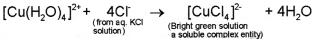Question 7.
What is the coordination entity formed when excess of aqueous KCN is added to an aqueous solution of copper sulphate? Why is it that no precipitate of copper sulphide is obtained when H2S(g) is passed through this solution?
Aqueous solution of copper sulphate contains Cu2+ ions in the form of complex entity, [Cu(H2O)4]2+ and H2O ligand is a weak ligand. When excess of KCN is added, a new coordination entity, [Cu(CN)4]2- is formed due to the following reaction: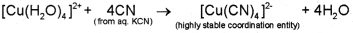Cyanide ligand, CN is a strong field lignad and stability constant of [Cu(CN)4]2- is quite large and thus practically no Cu2+ ions are left in solution. On passing H2S gas, no CuS is formed due to non- availability of Cu2+ ions in solution.

Question 8.
Optical isomerism is usually exhibited by complexes containing polydentate ligand. What do you mean by ligand?
Ligand is a neutral molecule or charged ion which can donate a lone pair of electron to the metal.

Question 9.
Coordination complexes are of different types. Name the compounds.

1. [Cr(H2O)5Cl2]
2. K3[Cr(C2O4)3]

2. Potassiumtrioxalatochromate(III)Question 10.
Write the IUPAC names of the following compounds.

1. K3M[Fe(CN)6]
2. [C0(NH3)5(CO3)]Cl

1. Potassiumhexacyanoferrate(III)
2. Pentaamminecarbanatocobalt(III) chloride

Question 11.
[Fe(CN)6]3- is paramagnetic, while [Fe(CN)6]4- is diamagnetic. Explain with the help of VB theory.
In [Fe(CN)6]3- iron is in +3 state and in [Fe(CN)6]4, iron is in +2 state. [Fe(CN)6]3- contains five electrons in d-level (3d5). In this complex iron undergoes d2sp3 hybridisation.

Due to the presence of one unpaired electron, [Fe(CN)6]3-, is paramagnetic. In [Fe(CN)6]4- iron contains six electrons in d-level (3d6). It undergoes d2sp3 hybridisation and has no unpaired electrons. Hence, [Fe(CN)6]4- is diamagnetic.

### Plus Two Chemistry Coordination Compounds Three Mark Questions and Answers

Question 1.
Look at the following two diagrams.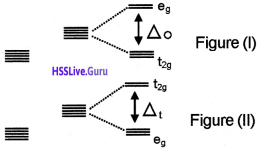1. Is the diagrams I and II correct? Justify. If the figure is not correct, redraw it.
2. Which theory is related to this?
3. Explain briefly, how this theory is applicable to octahedral complexes.

1. No, Figure (II) is wrong.2. Crystal field theory.

3. In the case of octahedral complexes, the ligands are approaching the ‘d’ orbitals through the axis. As a result of this the energy of dx²-y² and d orbitals increases and the energy of the remaining three orbitals decreases. The orbitals which possess high energy are represented as ‘eg’ levels and the orbitals which possess less energy are represented as “t2g” levels.Question 2.
A list of coordination compounds are given below:
[Cr(H2O)6] Cl3, [Co(NH3)5Br] SO4, [Co(NH3)5NO2]2+ and [Pt(NH3)2Cl2]. Which type of isomerism do these compounds exhibit?
Hydrate Isomerism, Ionisation Isomerism, Linkage Isomerism, Geometrical Isomerism.

Question 3.
The following are examples of coordination compounds. Identify the type of isomerism exhibited by each of them and write their possible isomers,

1. [Cr(NH3)5Br]SO4
2. CrCl3.6H2O
3. [PtCl2(NH3)2]

1. Cr(NH3)5Br]SO4 – Ionisation isomerism – [Cr(NH3)5SO4]Br
2. CrCl3.6H2O – Hydrate isomerism – [Cr(H2O)5Cl]Cl2.H2)
3. PtCl2(NH3)2] – Geometrical isomerism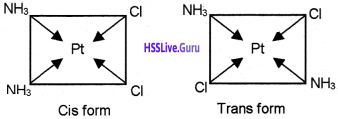Question 4.
What will be the correct order for the wavelengths of absorption in the visible region for the following:
[Ni(NO2)6]4-, [Ni(NH3)6]2+, [Ni(H2O)6]2+
The order of the ligand in the spectrochemical series is H2O < NH3 < NO2. Hence the wavelength of the light observed will be in the order:
[Ni(H2O)6]2+ < [Ni(NH3)6]2+ < [Ni(NO2)6]4-

Thus, the wavelength of light absorbed (E = $$\frac{\mathrm{hc}}{\lambda}$$) will be in the opposite order:
[Ni(H2O)6]2+ > [Ni(NH3)6]2+ > [Ni(NO2)6]4-

Question 5.
In a classroom discussion, Sajan argued that CN, OH, Cl etc. are examples for neutral ligands.

1. Do you agree with his argument?
2. If not, give a reason with the help of examples.
3. What do you mean by chelating ligand and chelation?

1. No.
2. They are charged ligands.
3. If a polydentate ligand is coordinated to the metals, a ring structure is obtained. It is called chelate and the phenomenon is called chelation.Question 6.
Ligands can be arranged according to the magnitude of Δ0 and the arrangement is given below:
l- < Br < Cl < F < OH < H2O < NH3 < (en)

1. What is this series known as?
2. Is the sequence incorrect order?
3. Identify the weak field and strong field ligands.

1. Spectrochemical series
2. Yes
3. The ligands above water are strong ligand and the ligands below water are weak ligands.

Weak filed ligands – l, Br, Cl, F, OH, H20 Strong filed ligands – NH3, en

Question 7.
Consider the following coordination compounds.

• [Co(NH3)5 Cl] SO4
• [Co(NH3)5 SO4]Cl

1. Write down the IUPAC name of these compounds.
2. Name the isomerism exhibited by these compounds.

1. The IUPAC name of compounds

• Pentaamminechloridocobalt(III) sulphate
• Pentaamminesulphatocobalt(III) chloride

2. Ionisation isomerism

Question 8.
Consider the following compounds:
[Co (NH3)5 NO2] Cl2 and [Co (NH3)5 ONO] Cl2

1. Identify the isomerism exhibited by these compounds.
2. Explain ionisation isomerism with example.

2. This form of isomerism arises when the counter ion in a complex salt is itself a potential ligand and can displace a ligand which can then become the counter ion.
e.g. [Co(NH3)5Cl] SO4 & [Co(NH3)5SO4] Cl

These complexes ionises as:

• [Co(NH3)5Cl] SO4 → [Co(NH3)5Cl]2+ + SO42-
• [Co(NH3)5SO4] Cl → [Co(NH3)5SO4]+ + Cl

Question 9.
Consider the statement: Crystal Field Theory (CFT) is applicable to octahedral and tetrahedral complexes.

1. Is this statement true?
2. Explain the crystal field splitting in octahedral complexes with the help of a neat diagram.

1. Yes
2. In an octahedral crystal field the ligands are approaching the metal along the axes. Hence, the energy of dx²-y² and d orbitals (eg set) increases by 3/5Δ0and that of dxy, dyz and dxz orbitals (t2g set) decreases by 2/5 Δ0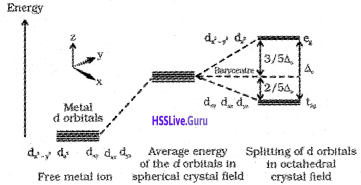Question 10.
When a ligand approaches to an octahedral complex, the degenerate ‘d’ orbitals undergoes splitting.

1. What will be the observation?
2. What are the factors influencing crystal field splitting energy?

1. The energy of dx²-y² and d orbitals (eg set) increases by 3/5Δ0 and that of dxy, dyz and dxz orbitals (t2g set) decreases by 2/5 Δ0

2. The factors influencing crystal field splitting energy.

• Nature of the ligand
• Geometry of the complex
• Valency of the metal

Question 11.
What will happen when a ligand approaches to a tetrahedral complex?
the energy of dxy, dyz and dxz orbitals (t2g set) increases by 2/5Δt and that of dx²-y² and d orbitals (eg set) decreases by 3/5 Δt.

Question 12.
Consider the complex ion [Ti (H2O)6]3+ In the case of an octahedral complex, what is the condition for the pairing of forth electron in the d- level?
If the crystal field splitting energy is greater than the pairing energy, the fourth electron will pair at the t2g level and if the pairing energy is greater than the crystal field splitting energy the electron will go to the on eg level.

Question 13.
Is bidentate ligands same as the amidentate ligands? Justify.
A bidentate ligand like (en), can form two coordinate bonds with the metal at the same time.
An amidentate ligand like -NO2 can form only one coordinate bond with the metal at a time. But it can ligate through two different atoms.

### Plus Two Chemistry Coordination Compounds Four Mark Questions and Answers

Question 1.
Match the following table: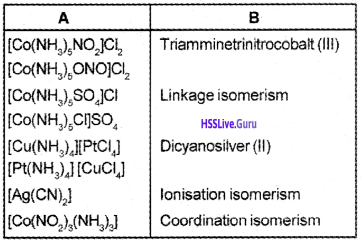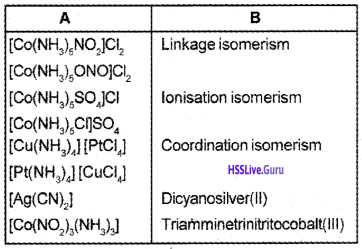Question 2.
1. Write the IUPAC names of the following coordination compounds.

• [Pt(NH3)Cl(NO2]2)
• K3[Cr(C2O4)3]

2. Identify the type of isomerism exhibited by the following complexes and distinguish them. [Co(NH3)5SO4] Br and [Co(NH3)5Br] SO4
1. The IUPAC names of the coordination compounds:

• Amminechloridonitrito-N platinum(II)
• Potassiumtrisoxalatochromate(III)

2. Ionisation isomerism.
The first compound gives pale yellow ppt. with AgNO3 solution whereas the second compound gives white ppt. with BaCl2 solution.

Question 3.
1. Write the IUPAC name of the following compounds:

• [Pt(NH3)2Cl2]
• K4[Fe(CN)6]

2. A list of coordination compounds are given below:

• [Cr(H2O)6]Cl3,
• [CO(NH3)5 Br]SO4,
• [CO(NH3)5 NO2]2+,
• [CO(NH3)6] [Cr(CN)6]

Which type of isomerism do these compounds exhibit?
1. The IUPAC name of the coordination compounds:

• Diamminedichloridoplatinum(II)
• Potassium hexacyanoferrate(II)

2. Type of isomerism

• [Cr(H2O)6]Cl3 – Hydrate isomerism
• [CO(NH3)5 Br]SO4 – Ionisation isomerism
• [CO(NH3)5 NO2]2+ – Linkage isomerism
• [CO(NH3)6] [Cr(CN)6] – Coordination isomerismQuestion 4.
1.. Write down the IUPAC name of

• K4[Fe(CN)6]
• [Pt(NH3)2Cl2]

2. On the basis of VBT, explain why [Fe(H2O)6]2+ is strongly paramagnetic while [Fe(CN)6]3- is weakly paramagnetic.
1. The IUPAC names are:

• Potassium hexacyanoferrate(II)
• Diamminedichloridoplatinum(II)

2.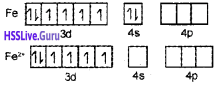In [Fe(H20)6]2-, iron undergoes sp3d2 hybridisation (inner orbital complex). It has four unpaired electrons and hence it is highly paramagnetic.In [Fe(CN)6]3+. iron undergoes d2sp3 hybridisation (outer orbital complex). It has only one unpaired electron. Hence, it is less paramagnetic.

Question 5.
The names of some co-ordination compounds are given below:

• EDTA
• Haemoglobin
• cis-platin
• Vitamin B12
• D-penicillamine
• Chlorophyll
• Ni(CO)4

a. Classify the above compounds on the basis of application of coordination compounds?
b. There are given some of the coordination compounds Name them.

1. K3[Fe(C2O4)3]
2. [Cr(CN)3]3+
3. [CoSO4(NH3)4]NO3
4. [CO(NO2)3(NH3)3]

a. On the basis of application of coordination compounds:

• In biological system – Haemoglobin, Vitamin B12, Chlorophyll.
• Estimation of hardness of water – EDTA.
• Extraction of metals – Ni(CO)4
• In medicine – D-penicillamine, cis-platin

b. The coordination compounds are:

1. K3[Fe(C2O4)3] – Potassiumtrioxalatoferrate(III)
2. [Cr(CN)3]3+ – Trisethylenediaminechromium(III) ion
3. [CoSO4(NH3)4]NO3 – Tetraamminesulphato- cobalt(III) nitrate
4. [CO(NO2)3(NH3)3] – Triamminetrinitrito-N-cobalt(III)

Question 6.
1. Name the following compounds.

• [Pt(NH3)4 ] [CuCl4]
• [PtCl2 (NH3)4] Br2

2. What type of isomerism is shown by the following coordination compounds?

• [Pt(NH3)4 ] [CuCl4]
• [Cr(en)3]3+

1.

• Tetraammineplatinum(II) tetrachlorocuprate(II)
• Tetraamminedichloridoplatinum(IV) bromide

2. Type of isomerism:

• Coordination isomerism
• Optical isomerismQuestion 7.

1. Write the d-orbital configuration of [Ti(H2O6]2+
2. Ti4+ is colourless. Why?
3. Write the possible isomers of [Co(NH3)5 Br] SO4 and name them.

1.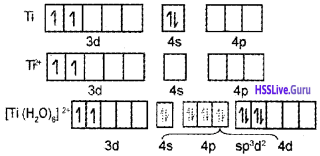2. In Ti4+ there are no electrons in 3d orbitals. Hence ‘d-d’ transition cannot take place. Hence it is colourless.

3. Possible isomers of [Co(NH3)5 Br] SO4 and names

• [CO(NH3)5 Br] SO4 – Pentamminebromido- cobalt(III) sulphate
• [Co(NH3)5SO4]Br-Pentamminesulphatecobalt(III) bromide

Question 8.
‘A’ and ‘B’ are isomers. They have the same composition. But ‘B’ cannot give the test for sulphate.

1. Write two suitable coordination compounds which give the test for sulphate.
2. What are the two major classes of isomerism exhibited by coordination compounds?
3. Draw the structure of an octahedral complex that show optical isomerism.

1. Two suitable coordination compounds which give the test for sulphate

• [CO(NH3)5Cl]SO4
• [Co(NH3)5Br] SO4

2. Structural isomerism, Stereoisomerism
3. [PtCl2(en)2]2+Question 9.

1. Write the formula of the complex ion Chloridonitro tetramminecobalt(III)?
2. Identify the ligands, coordination number and coordination sphere.
3. Explain the structure of Tetracarbonylnickel(O) with the help of Valence Bond Theory.

1. [CO(NH3)4Cl(NO2)
2. Ligands-NH3, Cl , NO2 Coordination number -4
3. Tetracarbonylnickel(0) – [Ni(CO)]4] – StructureNi(CO4) is diamagnetic as it does not contain any unpaired electron.

Question 10.
Consider the complex ion [Ti (H2O)6]3+

1. What is its outer electronic configuration and its shape?
2. What do you mean by crystal field splitting theory?

1. Ti3+ – 3d1 4s0 Octahedral
2. In the case of an isolated gasesous metal atom/ ion all the five d-orbitals have the same energy (degenerate). Due to the presence of ligands are splitted the degeneracy of the d-orbitals is lifted.Question 11.
Coordination compounds are those compounds which retain their identity even in solution and it is essential for all living matter.

1. Name a coordination compound containing Magnesium, which is essential for plants.
2. When we coordinate EDTAwith any metal, we get a ring structure. What is this process called?
3. Explain.

1. Chlorophyll.
2. Chelation.
3. When a poly dentate ligand is coordinated to the metal, a ring structure is obtained, called chelate complex. Such complexes are more stable than similar complexes containing unidentate ligands.

Question 12.
Some ligands are given below. Arrange them in suitable headings.
[H2O, NH3, CN, CO, Cl, OH (en)]
1. What do you mean by the term ligand?
2. Write down the nomenclature of the coordination compounds given below.

• K4[Fe(CN)6]
• [Ag (NH3)2]Cl

Neutral ligands – H2O, NH3, CO, en
Charged ligands – CN, OH, Cl
1. Ligand is a neutral molecule or charged ion which can donate atleast one lone pair of electron to the metal.
2. nomenclature of the coordination compounds

• Potassium hexacyanoferrate(II)
• Diamminesilver(I) chloride

Question 13.

1. What do you mean by optically active compounds? Give two examples.
2. Draw the ‘d’ and T forms of [Co(en)3]3+.

1. Optically active compounds are formed by chiral moneluces i.e., molecules which do not have plane of symmetry. These isomers are non- superimposable mirror images of each other. They are optically active and rotate the plane of polarised light equally but in opposite directions.

The isomer which rotates the plane of polarised light towards left is called leavorotatory (-) while that which rotate plane towards right is called dextrorotatory (+).
e.g. [Co(en)3]3+, [PtCl2(en)2]2+
Dextro and laevo forms of these compounds are possible.

2.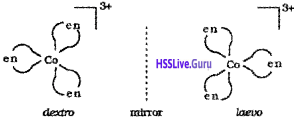Question 14.
What is the importance of the following coordination compounds is different fields?

1. EDTA
2. Gold cyanide [AU(CN)2]
3. cis-platin
4. [Ag(S2O3)]3-

1. EDTA → Estimation of hardness of water
2. AU(CN)2 → Metallurgy
3. cis-platin → Cancer therapy
4. [Ag(S2O3)]3- → Photography

Question 15.
The d-block elemetns forms coordination compounds.

1. Name the coordination compound K3 [CoF6].
2. Write the electronic configuration of the central metal atom of the above complex by using CFT
3. Draw the figure to show the splitting of degenerate, ‘d’ orbitals in an octahedral field.

1. K3[CoF6] – Potassiumhexafluridocobaltate(III)
2. The electronic configuration of Co (Z = 27) is [Ar]3d74s2 In K3 [CoF6], Co is in +3 state. The configuration of Co3+ is 3d6 4s°.
3.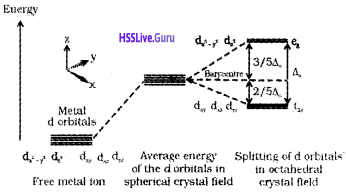Question 16.

1. Name the compound K3[Cr(C2O4)3]
2. Explain on the basis of VB theory that [Ni(CN)4]2- ion with square planar structure is diamagnetic and [Ni(Cl)4]2- ion with tetrahedral structure is paramagnetic.

1. K3[Cr(C2O4)3] – Potassiumtrioxalatochromate(III)
2. Ni = 1s²2s²2p63s²3p64s²3d²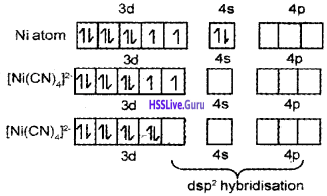It is diamagnetic due to absence of unpaired electrons.∴ Due to presence of unpaired electrons it is paramagnetic.

### Plus Two Chemistry Coordination Compounds NCERT Questions and Answers

Question 1.
FeSO4 solution mixed with (NH4)2SO4 solution in 1:1 molar ratio gives the test of Fe2+ ion but CuSO4 solution mixed with aqueous ammonia in 1:4 molar ratio does not give the test of Cu2+ ion. Explain why?
FeSO4 does not form any complex with (NH4)2SO4. Instead, it forms a double salt FeSO4.(NH4)2SO4.6H2O which dissociates completely into ions. CuSO4 when mixed with NH3 forms a complex [CU(NH3)4]SO4 in which the complex ion [CU(NH3)4]2+ does not dissociate to give Cu2+ ion.

Question 2.
Write the geometrical isomers of [Pt(NH3)(Br)(Cl) (py)] and how many of these will exhibit optical isomerism?
The complex [Pt(NH3)(Br)(Cl)(py)] will form three geometrical isomers:Square planar complexes of this type will not show geometrical isomerism.Question 3.
Aqueous copper sulphate solution (blue in colour) gives:

1. a green precipitate with aqueous potassium fluoride and
2. a bright green solution with aqueous potassium chloride. Explain these experimental results.

Aqueous copper sulphate contains coordination entity, [Cu(H2O)4]2+ which is blue in colour. Water molecule is a weaker ligand than Cl and F.
1. On addition of aqueous KF solution, a new complex entity is formed wich is green in colour.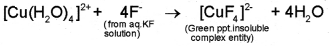2. On addition of aqueous solution of KCl, another bright green complex entity is formed which is soluble in water.Question 4.
What is the coordination entity formed when excess of aqueous KCN is added to an aqueous solution of copper sulphate? Why is it that no precipitate of copper sulphide is obtained when H2S(g) is passed through this solution?
Aqueous solution of copper sulphate contains Cu2+ ions in the form of complex entity, [Cu(H2O)4]2+ and H2O ligand is a weak ligand. When excess of KCN is added, a new coordination entity, [Cu(CN)4]2- is formed due to the following reaction:Cyanide ligand, CN is a strong field lignad and stability constant of [Cu(CN)4]2- is quite large and thus practically no Cu2+ ions are left in solution. On passing H2S gas, no CuS is formed due to non-availabiliy of Cu2+ ions in solution.Question 5.
What will be the correct order for the wavelengths of absorption in the visible region for the following:
[Ni(NO2)6]4-, [Ni(NH3)6]2+, [Ni(H2O)6]2+
Thus, the wavelength of light absorbed (E = $$\frac{\mathrm{hc}}{\lambda}$$) will be in the opposite order: